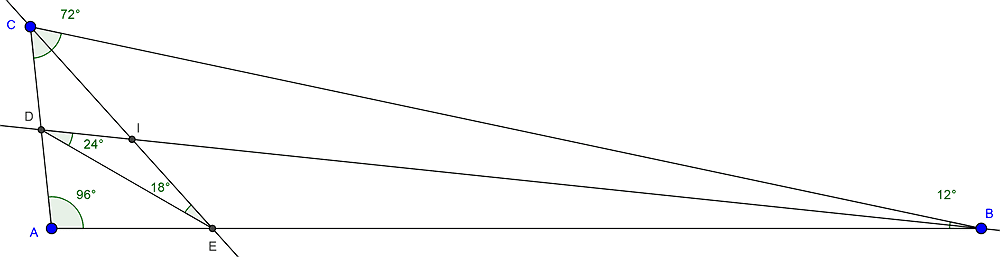# Difference of angles in a triangle

• MHB
• maxkor

#### maxkor

In a triangle ABC, let D and E be the intersections of the bisectors of the angles ABC and ACB with the sides AC and AB, respectively. Knowing that the measures in degrees of the angles BDE and CED are equal to 24 and 18, respectively, calculate the difference in degrees between the measures of the two smallest angles of the triangle. CAB angle = 96 degrees.

I checked with Geogebra:But how to solve this problem without Geogebra?

Recalling that plane Euclidean triangle angles add up to 180 degrees, opposite angles in intersecting lines are equal and angle bisectors split angles in half, we can use inspection to determine all the angles in the diagram.

As an example, by inspection, triangle DEI has two angles shown so we can compute the missing angle EID as 180-24-18 = 138 degrees.

•Greg Bernhardt
so how to calculate for example angle ABC?

•malawi_glenn and jedishrfu